7811 106 1

# How To Test Bids In PPC Advertising Without Applying ThemAndrey Belousov
Growth Hacker at Serpstat
A/B tests are an integral part of any marketing strategy because you can increase its effectiveness in several times. In contextual advertising such testing also takes place, for example, to create a high-quality, attractive ad. But are they really that effective? Let's deal with them, and also find out another secret of successful testing.
A/B testing problems
A/B tests of bids have several obstacles:
1
They require a huge number of clicks for reliable results.
2
Almost always, bids are tested only on the part of ad campaigns. The success of one variant over the other on the part of the account doesn't mean its advantage on the entire account.
3
Unlike testing changes on a website, it is not enough to simply compare the conversion rate of two options. You need to align the budget or CPA or CRR and compare the number of conversions or revenue. However, the alignment of budget and CPA occurs with some margin of error.
In general, even with an infinite amount of data, we will be able to determine which option is better only if the options have a difference in the efficiency of more than 3-5%. Let's call this theoretical sensitivity.

The following factors influence theoretical sensitivity:
We don't test the entire account, but a specific part of it. Moreover, the keywords we test are not randomly selected.
We align the budget / CPA / CRR for the two options with some margin of error.
Checkerboard order is not the same as randomly distributing clicks between options.
In practice, since we don't have an infinite amount of data, even for large sites, the sensitivity threshold of the A/B test is about 10-20%. If the difference in effectiveness between the two options is less than this threshold, then doing A/B is not much better than flipping a coin. Let's call this practical sensitivity.

As a result, A/B testing:
• is not accurate;
• takes much time;
• requires a lot of work and specific knowledge;
• cannot be fully automated.

And after all, there is always a possibility that new rates will work worse.
How To Discover A Competitor's Budget For Contextual Advertising: 3 Unusual Methods
Equal Bid Improvement Factor
Therefore, there may be a metric that is more sensitive (even theoretical) than the A/B test. Moreover, this metric can be simply calculated using historical data without taking into account bids. If we automate this, then it can be done in a couple of clicks.

However, since we don't know the dependence of the number of clicks and CPC on the rate, then this metric will produce quantitative results with a rather large margin of error, but possibly quite accurate qualitative results.

In other words, this metric will answer the question "Which option is better?" Rather well, but rather poorly on the question "How much better?".
Intermediate calculations may seem voluminous to you, but the final formula will be short.

For the sake of simplicity, let's assume that each keyword has:
CPC and number of clicks are proportional to the bid;
conversion probability is independent of the bid.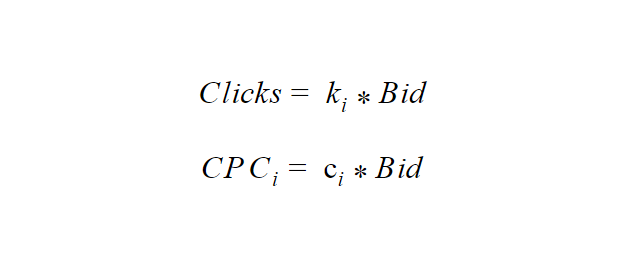Let's set the bids in proportion to the estimate: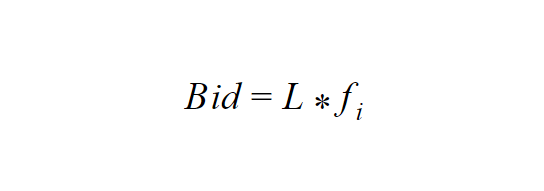Where:
f - forecast the probability of conversion
L is a constant to fit our constraints (budget, target CPA)

Let p be the probability of conversion, then the expected number of conversions is: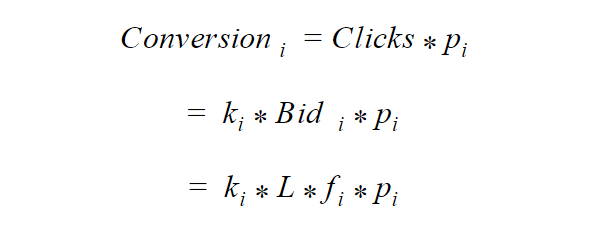The expenditure for a keyword is: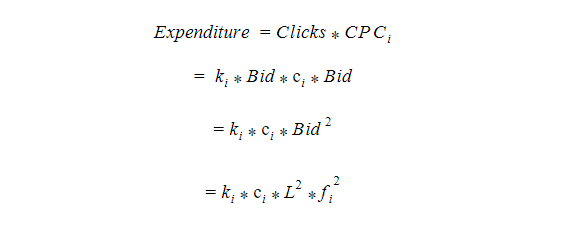Let our goal be the maximum conversions with a fixed CPA = TargetCPA.
The expected CPA is: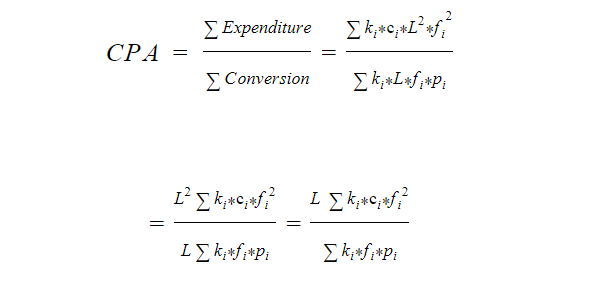To find L, let's equate the target CPA with the expected one: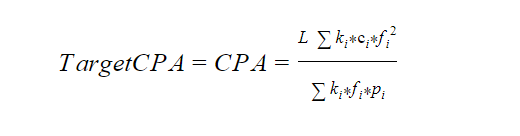From here you can calculate L: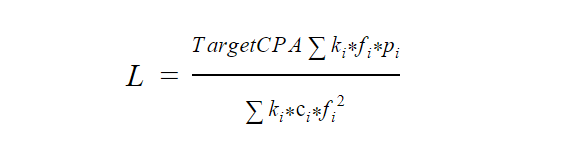We introduce the weighted mean operator: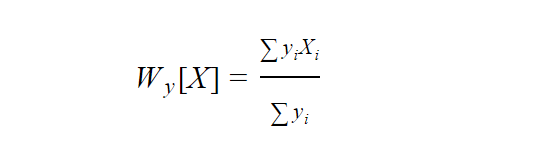From which it follows that: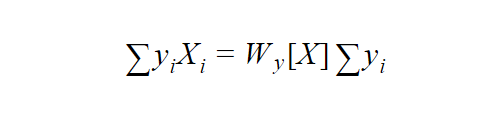You can also easily see that the constant can be taken out of the weighted mean: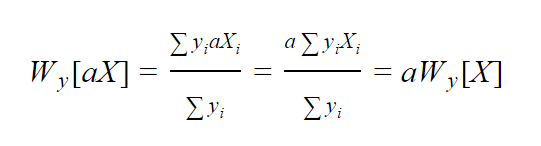Let's get back to L: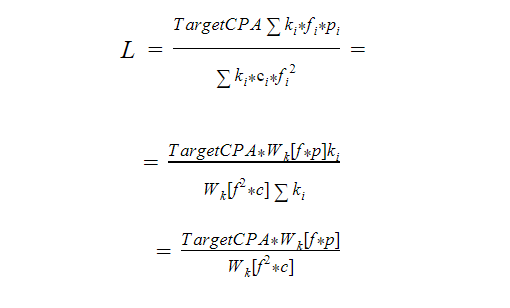For simplicity, we will assume that c and f are not correlated.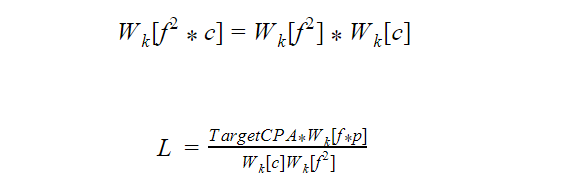Let's calculate the expected number of conversions: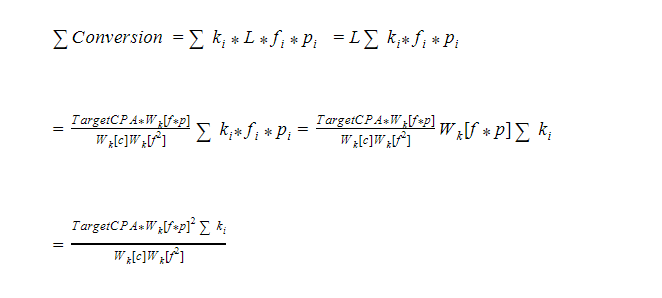Let's calculate what will happen at equal rates. Let our forecast for all keys be the same and equal to some constant m. Without loss of generality, we can say that m is equal to the weighted average conversion: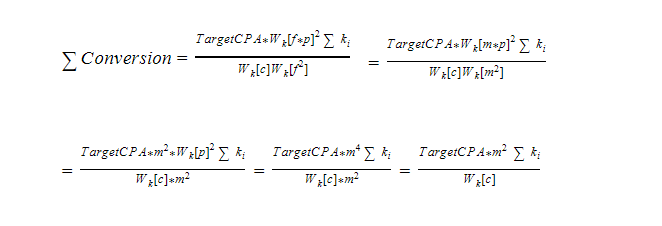Now let's divide the number of conversions for arbitrary bids by the number of conversions for equal bids. Let's get how many times bids bring more conversions than equal bids for the same CPA. Let's call this metric the Equal Bid Improvement Factor (IBIF).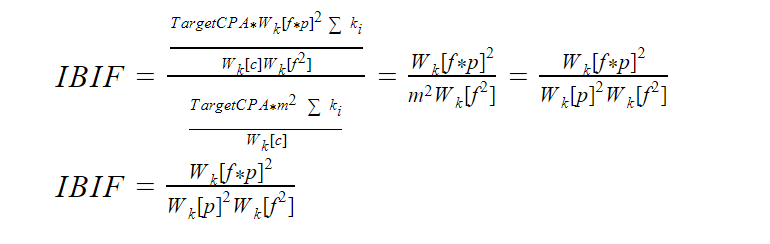With equal rates, IBIF should be equal to 1. For example, all rates are equal to the constant S: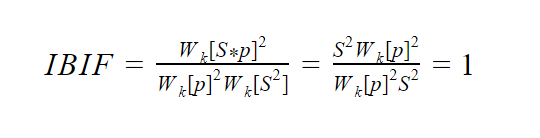W [p] is just a multiplier that doesn't depend on the estimate.
W [fp] - grows with an increasing correlation between the estimate and the probability of conversion. It also grows with bid variance (spread).
W [ff] - grows with increasing variance of bids.

IBIF
can be expressed in terms of rate variance and the correlation between bids and estimates.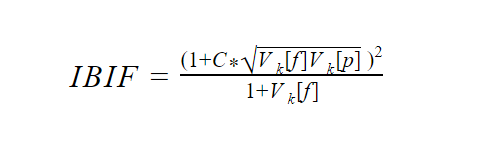Where:

C is Pearson's weighted correlation coefficient between bids and predictions. The measure of the adequacy of the bids doesn't exceed 1. Equal to 1 for an ideal estimate, equal to 0 for random rates.
V [] - weighted relative variance, the measure of the spread. Greater than or equal to zero. For example, if V [f] = 0, it means that all bids are the same. V [p] <1 is almost certainly true. Usually V [p] is about 0.5. V [f] is usually not very different from V [p].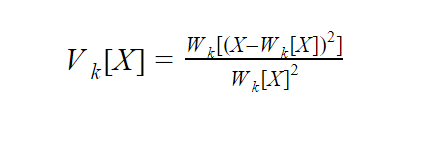If R[p] = 0.5: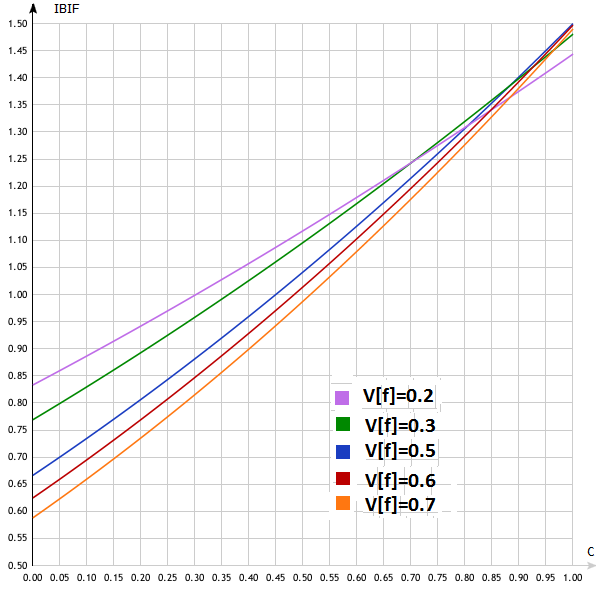It can be seen that, in general, the larger V [f], the stronger the IBIF depends on the correlation. It is also easy to see that at high correlations (more than 0.95), the IBIF is practically independent of V [f].
IBIF at ideal bids
At ideal bids, f=p, C=1: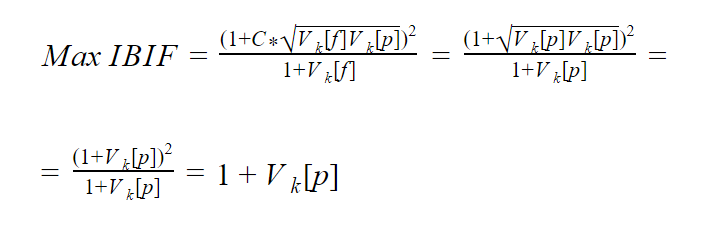The more different the conversions for keywords, the higher the IBIF can be obtained with an ideal estimate.

From the fact that it is almost certain that V [p] <1, we get that the IBIF is almost always less than 2. The opposite indicates, most likely, that a significant number of keywords have almost zero conversion (much less than the average for the site).

This can happen, for example, when you buy most of the clicks on products you don't have in stock, on 404 pages, or irrelevant pages; or some of the pages don't have a conversion tracking code.

In other words, IBIF > 2 may indicate that rates solve some specific problem.
How To Launch A Successful Advertising Campaign: 10 Lifehacks of API Console
Personal demonstration
Our specialists will contact you and discuss options for further work. These may include a personal demonstration, a trial period, comprehensive training articles, webinar recordings, and custom advice from a Serpstat specialist. It is our goal to make you feel comfortable while using Serpstat.
Finding IBIF from historical data
You can calculate IBIF from historical data using the formula: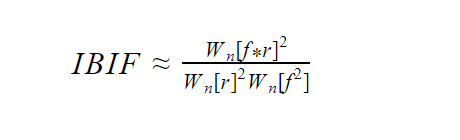Where:

r - keyword conversion rates
n - number of clicks on keywords

Note that f should not directly depend on r. That is, f and r must be calculated at different periods.

In other words, you need to run the test like this:
For example, on the 1st day we withdraw bids or calculate the forecast. Remember f.
After a couple of weeks, for example on the 16th, we take data from the 2nd to the 15th. And we get n and r.
We calculate the IBIF, the variant with the higher IBIF is considered the winner.
Since we don't apply bids, we just look at the statistics, we will call this test passive.

If we multiply f by some constant factor S that is the same for all keywords, then the IBIF will not change: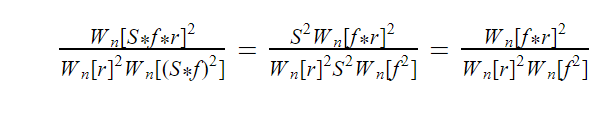Therefore, instead of f, you can take not only the forecast but also a set of rates that are proportional to the original estimates. That makes it possible to compare not only forecasts but also sets of bids with each other or a set of bids with a forecast.
Maximum IBIF
You can give the following assessment of the maximum IBIF (with an ideal forecast):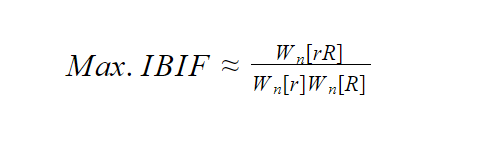Where:

r - conversion rate for the first period (regular rates are considered in this period)
n - number of clicks in the first period
R - conversion rate for the second period (periods should not overlap)

If you don't have a second period, then you can divide the first period into 2 parts (equal) then:

r - conversion rate in the first part
R - conversion rate in the second part
n - number of clicks for the entire period

This formula doesn't depend on uniform seasonality when the conversion of all keywords decreases or increases by the same number of times.

The accuracy of calculating the limiting IBIF for small data is several times less than that of a conventional IBIF.
2 Hours → \$ 38 → 41 Conversions: How To Create An Advertising Campaign Quickly And Safely
We have examined the problem "maximum number of conversions with a fixed CPA". However, IBIF can be applied to almost all tasks.
Maximum turnover
If we face the task of "maximum turnover with a fixed CRR", then the IBIF formula will not change. You just need to substitute the ratio of the turnover to the number of clicks instead of r.

However, the rule V [p] <1 and IBIF <2, in this case, may not be observed.
Fixed budget
If we have a fixed budget, then after calculations we get: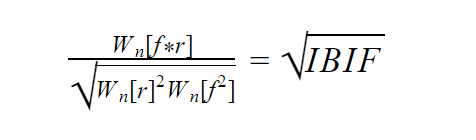Which will not lead to qualitative changes. If one forecast has a higher IBIF, then it has a higher root from the IBIF.
Bid modifiers
You can similarly calculate the IBIF not only for bids but also for bid modifiers by time of day, day of the week, and so on.

Seasonality accounting can also be evaluated.
Comparison
To compare 2 sets of bids or forecasts, you need to calculate this parameter: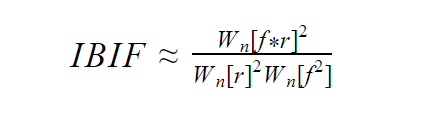Where:

r - keyword conversion rates or revenue-to-click ratio
n - number of clicks on keywords
f - bids or forecast for conversion or revenue per click. When calculating them, data for the period for which r was taken should not be used.
W [] average weight operatorEqual bids give IBIF = 1. The maximum IBIF can be calculated as follows: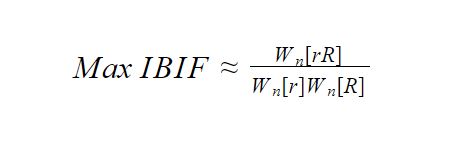Where R is the conversion rate of keywords or the ratio of revenue to clicks for another period that does not overlap with the first.
How To Optimize Contextual Advertising: Tricks And Lifehacks
Calculation in Excel
To calculate this indicator in Excel, first you need to get rid of the weighted mean.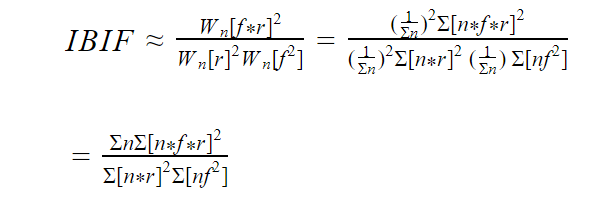Where:

n (clicks) are in column A
r (conversion) are in column B
f (forecast/bids) are in column C

Then the formula will look like this:
SUM(A1:A)*POWER(SUMPRODUCT(A1:A,B1:B,C1:C),2)/(POWER(SUMPRODUCT
(A1:A,B1:B),2)*SUMPRODUCT(A1:A,C1:C,C1:C))
However, the formula can be simplified if column D contains conversions and revenue:
SUM(A1:A)*POWER(SUMPRODUCT(C1:C,D1:D),2)/(POWER(SUM(D1:D),2)*
SUMPRODUCT(A1:A,C1:C,C1:C))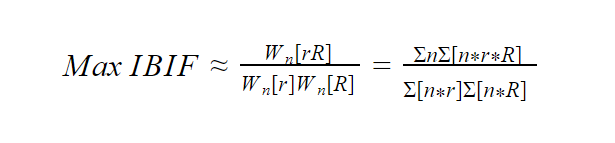Let R be in column G. Then the formula is:
SUM(A1:A)*SUMPRODUCT(A1:A,B1:B,G1:G)/(SUMPRODUCT(A1:A,B1:B) *SUMPRODUCT(A1:A,G1:G))
How To Collect A Semantic Core For Contextual Advertising And Get Maximum Outreach

### Speed up your search marketing growth with Serpstat!

Keyword and backlink opportunities, competitors' online strategy, daily rankings and SEO-related issues.The opinion of the guest post authors may not coincide with the opinion of the Serpstat editorial staff and specialists.

Rate the article on a five-point scale

The article has already been rated by 4 people on average 4 out of 5
Found an error? Select it and press Ctrl + Enter to tell us

## Discover More SEO Tools

Keywords Grouping

Unlock the power of your target audience's searched keywords with our keyword trends

Keyword Volume Checker

Bulk Keyword Volume Checker – get valuable information about your target keywords

Tools for Keywords

Keywords Research Tools – uncover untapped potential in your niche

### Recommended posts

Are you sure?

Introducing Serpstat

Find out about the main features of the service in a convenient way for you!

Please send a request, and our specialist will offer you education options: a personal demonstration, a trial period, or materials for self-study and increasing expertise — everything for a comfortable start to work with Serpstat.

Name

Email

Phone